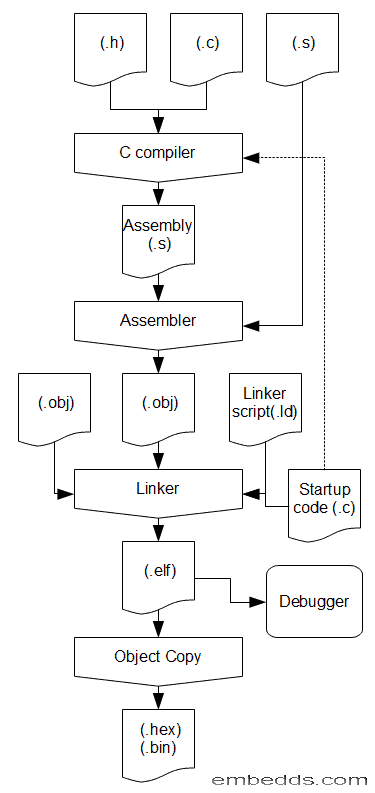# Programming STM32-Discovery using GNU tools. Linker script

Previously we went through setting up a development environment for ARM Cortex-M3 microcontroller. We decided that two equal choices will do the same job – either CodeSourcery G++ Lite or Yagarto. Both use the same base of GNU tool-set.

## Developing with GCC tools

To get a working binary, there is a series of tools involved during code development. Several tools are necessary to compile simple applications. These are compiler, assembler, linker and binary generator. Each of them does its task in a chain process. When you start compiling your project, usually there is a linker invoked, which with correct parameters links libraries, object files.Once executable is generated, then the binary image generator creates a binary image (.bin or .hex) that can be uploaded to MCU. We won’t go into details right now as this will be more convenient to do in later code examples. Let us get directly to code writing part which is very important for linker and tasks before main() routine.

Practically speaking, linker script is a file that defines microcontroller specific features like memory map, memory sections, stack location, and size. It may also contain application-specific information if needed. Usually, linker scripts are already written and can be found along with example projects. So once set up, there is no need to write them each time a new project is started unless some modifications or additions are needed. Let us look at some key features of the linker script.

First of all, we need to describe memory blocks and the size of the microcontroller. There is a MEMORY command used. Further, we are going to work with STM32 Discovery kit where STM32F100RB microcontroller is used which has 128KB of Flash and 8KB of SRAM. So first, we define our memory types:

```MEMORY
{
RAM (rwx) : ORIGIN = 0x20000000, LENGTH = 8K
FLASH (rx) : ORIGIN = 0x8000000, LENGTH = 128K
}```

Where “rwx” means rewritable and executable, “rx” – read-only and executable memory. Executable is meant that code can be run from this memory. In ARM program can be executed directly from Flash or RAM. ORIGIN points to start address of memory region and Length defines the size of particular memory type.

Each memory type is also divided into memory sections where different types of data are stored. One section is needed for variables, another for constants, code, stack, heap, and so on. So we need to show linker how to divide memories into the section. For this command, SECTIONS is used. First of all, we need to define the section where the program code will be stored. This section is called .text:

```SECTIONS
{
.text :
{
. = ALIGN(4);
KEEP(*(.interrupt_vector))
*(.text)
*(.text*)
*(.rodata)
*(.rodata*)
. = ALIGN(4);
} > FLASH```

Here. = ALIGN(4); indicates that section is aligned to the 4 byte boundary; KEEP(*(.interrupt_vector)) Means that for this section optimization must be skipped during linking; .text indicates program code section; .rodata – space for storing constants; >FLASH means that sections are located in FLASH memory. You can add even more sections if needed. The dot indicates the current address. So next we need to remember last used address by assigning this to the variable:

 1 `_data_flash = .;`

Now we can continue with next section – .data. This section usually is located in RAM and contains static data when variables have initially defined values.

 1 2 3 4 5 6 7 8 9 `.data : AT ( _data_flash )` `{` `. = ALIGN(4);` `_data_begin = .;` `*(.data)` `*(.data*)` `. = ALIGN(4);` `_data_end = .;` `} > RAM`

Here we are using saved flash address AT ( _data_flash ) where we can find initial constants stored in a flash that has to be loaded to RAM. This will be done by the startup code. For now, we create .data location where our constants will be loaded.

Next section is .bss. This is where undefined/uninitialized variables and globals will be stored.

```.bss :
{
_bss_begin = .;
__bss_start__ = _bss_begin;
*(.bss)
*(.bss*)
*(COMMON)
. = ALIGN(4);
_bss_end = .;
__bss_end__ = _bss_end;
} > RAM```

.bss section usually goes right after .data section.

And finally, we need to define stack.

 1 2 3 4 5 6 7 8 `_stack_size = 1024;` `_stack_end = ORIGIN(RAM)+LENGTH(RAM);` `_stack_begin = _stack_end - _stack_size;` `. = _stack_begin;` `._stack :` `{` `. = . + _stack_size;` `} > RAM`

Stack size can be changed or even expanded to the end of RAM. But then linker won’t have a chance to warn about a shortage of stack memory. Anyway, there are lots of variants of defining sections so we won’t go much into details.

The last thing to tell linker is where the program has to start after reset. So we use a simple line:

 1 `ENTRY(handler_reset);`

This means that before the main() program, we need to initialize variables – copy constants from Flash to RAM, initialize stack and do other stuff if needed. Initialization is done in startup code. This part will be left for another post. Right now, we’ve done the following:I won’t give final working linker script as it must go along with start-up code which will be discussed next.

1. Tectu

Hello,

You have a little mistake in the stack section:

Line 2 in the second last codebox is:

stack_end = ORIGIN(RAM) LENGTH(RAM);

but should be:

_stack_end = ORIGIN(RAM) LENGTH(RAM);

(underline in fromt of the stack_end).

2. wisementor

Not just CodeSource G but also Code::Blocks has capability to compile your code using GCC. For debugging you can use EPS debugger plugin for STM32.

3. stack_end = ORIGIN(RAM) LENGTH(RAM); changed to
_stack_end = ORIGIN(RAM) LENGTH(RAM);